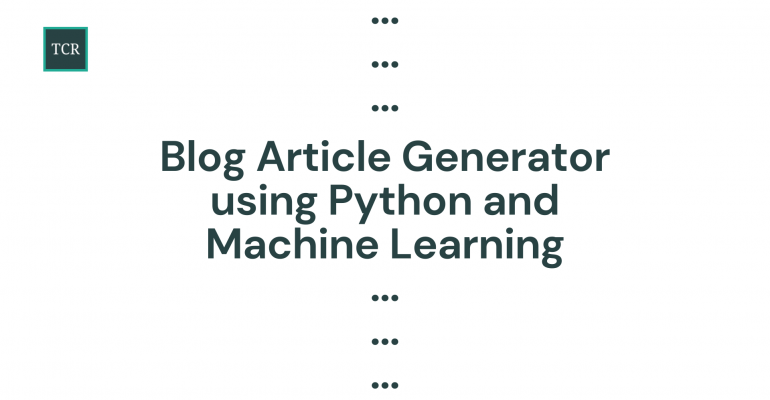Thanks to theidioms.com## Automatic Blog Article Generator using Python and Machine Learning# Automatic Blog Article Generator using Python and Machine Learning

Learn to perform text generation for creating automatic blog posts using the GPT-2 pre-trained model in just 3 lines of code with Python.

GPT-2 is a large transformer-based Machine Learning model created by OpenAI with 1.5 billion parameters and trained on a dataset of 8 million web pages. It is trained with a simple objective: predict the next word, given all of the previous words within some text.

First, let us install the `transformers` library from Hugging Face for using GPT-2,

`pip install transformers`

Next, importing the `pipeline` function from the transformers library,

```# Importing the pipeline function from the transformers library
from transformers import pipeline```

The pipeline method is responsible for:

• Pre-processing: Converting raw text input to numerical input for the pre-trained GPT-2 model
• Model Inference: Making a prediction using the pre-trained GPT-2 model
• Post-processing: Converting prediction to a proper output

Calling the pipeline function by specifying the task as ‘text-generation’ and model as ‘gpt2’,

```# Creating a TextGenerationPipeline for text generation

Note: The `gpt2` pre-trained model is large in size so it will take some time to download.

Now, the final step is to give a starting phrase or sentence to the `generator` pipeline and let the model generate relevant text of specified length.

```# Generating
generator("It takes time to write a good blog post.", max_length=60, num_return_sequences=5)```

You can change the value of `max_length` and `num_return_sequences` to specify how long you want the generated text to be and how many return sequences should be generated respectively.

The full code is as follows:

```# Importing the pipeline function from the transformers library
from transformers import pipeline

# Creating a TextGenerationPipeline for text generation

# Generating
generator("It takes time to write a good blog post.", max_length=60, num_return_sequences=5)```

Build your data skills online by learning Python, SQL, R, Excel, and much more: Join Datacamp!